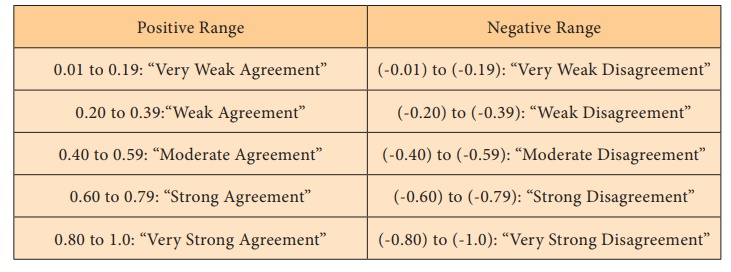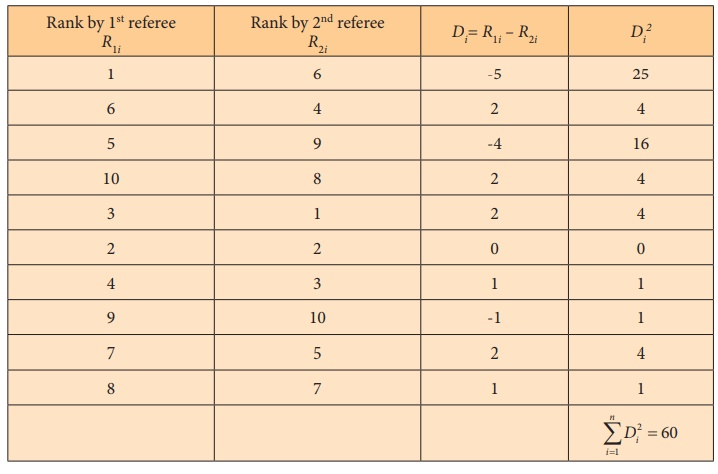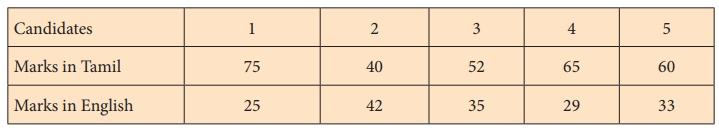Home | | Statistics 12th Std | SpearmanŌĆÖs Rank Correlation Coefficient

# SpearmanŌĆÖs Rank Correlation Coefficient

If the data are in ordinal scale then SpearmanŌĆÖs rank correlation coefficient is used. It is denoted by the Greek letter Žü (rho).

SPEARMANŌĆÖS RANK CORRELATION COEFFICIENT

If the data are in ordinal scale then SpearmanŌĆÖs rank correlation coefficient is used. It is denoted by the Greek letter Žü (rho).

SpearmanŌĆÖs correlation can be calculated for the subjectivity data also, like competition scores. The data can be ranked from low to high or high to low by assigning ranks.

SpearmanŌĆÖs rank correlation coefficient is given by the formulawhere Di = R1i ŌĆō R2i

R1i = rank of i in the first set of data

R2i = rank of i in the second set of data and

n = number of pairs of observations

## Interpretation

SpearmanŌĆÖs rank correlation coefficient is a statistical measure of the strength of a monotonic (increasing/decreasing) relationship between paired data. Its interpretation is similar to that of PearsonŌĆÖs. That is, the closer to the ┬▒1 means the stronger the monotonic relationship.Example 4.3

Two referees in a flower beauty competition rank the 10 types of flowers as follows:Use the rank correlation coefficient and find out what degree of agreement is between the referees.

Solution:Interpretation: Degree of agreement between the referees ŌĆśAŌĆÖ and ŌĆśBŌĆÖ is 0.636 and they have  ŌĆ£strong agreementŌĆØ in evaluating the competitors.

Example 4.4

Calculate the SpearmanŌĆÖs rank correlation coefficient for the following data.Solution:Interpretation: This perfect negative rank correlation (- 1) indicates that scorings in the subjects, totally disagree. Student who is best in Tamil is weakest in English subject and vice-versa.

Example 4.5

Quotations of index numbers of equity share prices of a certain joint stock company and the prices of preference shares are given below.Using the method of rank correlation determine the relationship between equity shares and preference shares prices.

Solution:Interpretation: There is a negative correlation between equity shares and preference share prices.

There is a strong disagreement between equity shares and preference share prices.

## Repeated ranks

When two or more items have equal values (i.e., a tie) it is difficult to give ranks to them. In such cases the items are given the average of the ranks they would have received. For example, if two individuals are placed in the 8th place, they are given the rank [8+9] / 2 = 8.5 each, which is common rank to be assigned and the next will be 10; and if three ranked equal at the 8th place, they are given the rank [8 + 9 +10] /3  = 9 which is the common rank to be assigned to each; and the next rank will be 11.

In this case, a different formula is used when there is more than one item having the same value.where mi is the number of repetitions of ith rank

### Example 4.6

Compute the rank correlation coefficient for the following data of the marks obtained by 8 students in the Commerce and Mathematics.### Solution:Repetitions of ranks

In Commerce (X), 20 is repeated two times corresponding to ranks 3 and 4. Therefore, 3.5 is assigned for rank 2 and 3 with m1=2.

In Mathematics (Y), 30 is repeated three times corresponding to ranks 3, 4 and 5. Therefore, 4 is assigned for ranks 3,4 and 5 with m2=3.

Therefore,Interpretation: Marks in Commerce and Mathematics are uncorrelated

Tags : Repeated ranks, Example Solved Problems , 12th Statistics : Chapter 4 : Correlation Analysis
Study Material, Lecturing Notes, Assignment, Reference, Wiki description explanation, brief detail
12th Statistics : Chapter 4 : Correlation Analysis : SpearmanŌĆÖs Rank Correlation Coefficient | Repeated ranks, Example Solved Problems